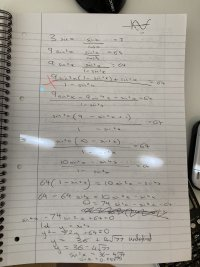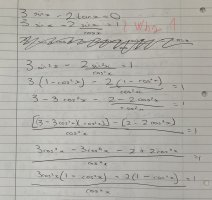# Solving trigonometry questions

#### lanaaaaaa8

##### New member
Hello everyone,

I hope you’re all well today. I study A Level mathematics (roughly college level mathematics in the US) in the UK and I have some questions regarding trigonometric identities. I have basically been trying to figure out the answers to some solve questions and I am either unsure whether what I have done is correct ( the answers for this worksheet I am doing are extremely unclear), or I have got stuck and I am unsure how to proceed.

I have attached pictures of my work with the questions below. I have tried to put the question first and my subsequent working out in the next photo.

Please note that these questions are ‘solve’ questions.

I would be extremely grateful for any help, as I have been working on identities for a few days now and I am feeling quite disheartened at the number of questions I cannot seem to do.

#### Harry_the_cat

##### Elite Member
With Question 1, your first step to write tan x as (sin x)/(cos x) was a good start.
Go back to that step and multiply both sides by cos x.
Then replace the sin^2 x with an expression involving cos^2 x.
Can you see what to do know?

#### Harry_the_cat

##### Elite Member
For Q2, again first step is good.
Not sure how you got the third line. That's the same as saying $$\displaystyle 3a-2b=1$$ means that $$\displaystyle 3a^2-2b^2=1$$ which isn't true.

Try factorising out the sin x from your second line and then use the null factor law.

Can you proceed?

#### Zermelo

##### Junior MemberThese are your mistakes buddy, but it was pretty close! In the first question, you added the terms instead of multiplied. In the other, a magic 1 appearedListen to Harry, and try not to complicate! I wouldn't square both sides in either of these questions...
Try to boil it all down to a single trigonometric function, such that you only work with sines or cosines...

#### Harry_the_cat

##### Elite Member
Oh yeah I missed the 0 suddenly changing to a 1. Should remain 0 (which is why the null factor law will be appropriate).

#### canvas

##### New member
It's pretty simple dude, check out my solution!

$3\sin(x)-2\tan(x)=0, x\neq\frac{\pi}{2}+k\pi, k\in\Z\\3\sin(x)-\frac{2\sin(x)}{\cos{x}}=0\\\sin(x)(3-\frac{2}{\cos(x)})=0\\\sin(x)=0\vee \frac{2}{\cos(x)}=3 \Rightarrow \cos(x)=\frac{2}{3}\\\\x=k\pi, k\in\Z \vee x=\pm\arccos(\frac{2}{3})+2k\pi, k\in\Z$

#### canvas

##### New member
$3\sin(x)\cdot\tan(x)=8,\,\,x\neq\frac{\pi}{2}+k\pi, \,\,k\in\Z\\3\sin(x)\cdot\frac{\sin(x)}{\cos(x)}=8 /\cdot\cos(x)\\3\sin^2(x)=8\cos(x)\\\\3[1-\cos^2(x)]=8\cos(x)\\3-3\cos^2(x)=8\cos(x)\\Let\,\,t=\cos(x), \,t\in[-1,1]\\3t^2+8t-3=0\\(3t-1)(t+3)=0\\t=\frac{1}{3}\,\vee\,t=-3\notin[-1,1]\\\cos(x)=\frac{1}{3} \Rightarrow x=\pm\arccos(\frac{1}{3})+2k\pi,\,\,k\in\Z$

#### Harry_the_cat

##### Elite Member
Hi Canvas, beautiful worked solutions, but... On this site, however, we try to help the poster reach their own solutions, by showing them where they've gone wrong and giving them hints to get on the right track. Please remember that for next time. Not meaning to devalue your work, but any of the helpers here could have provided the fully worked solutions you have provided.
Please continue contributing, you obviously have a good understanding of trigonometry, and I'm sure other areas of mathematics.

•topsquark and Subhotosh Khan